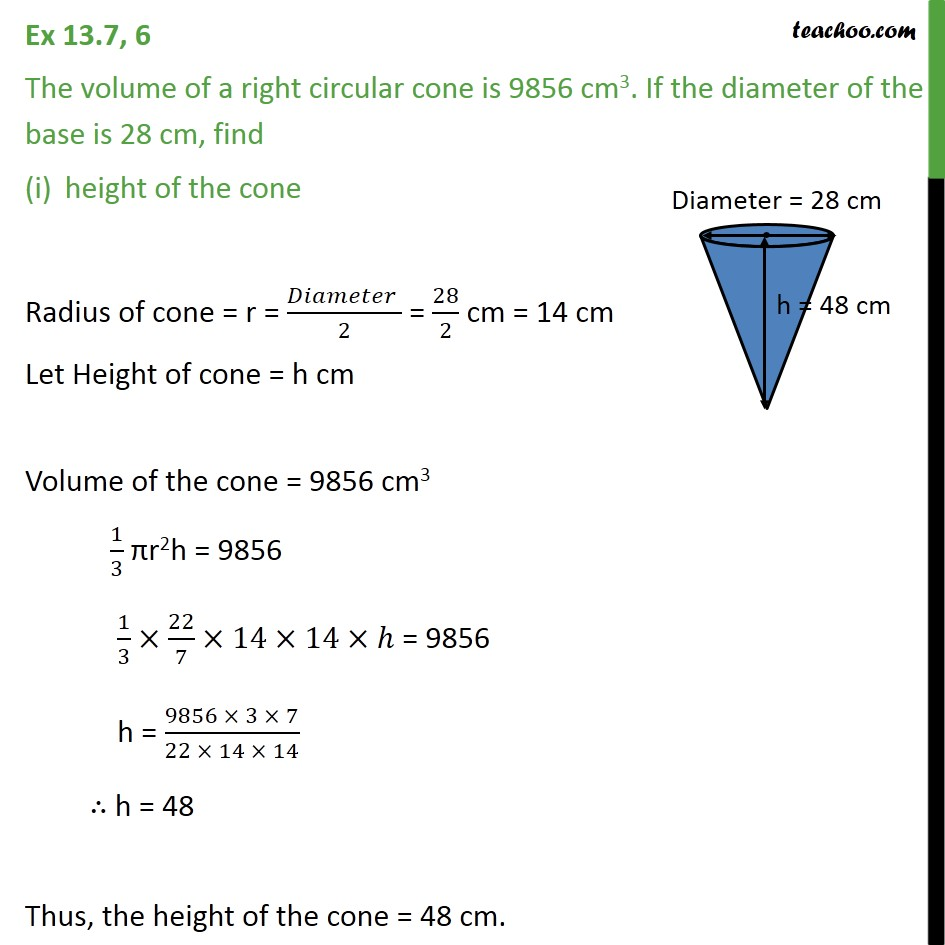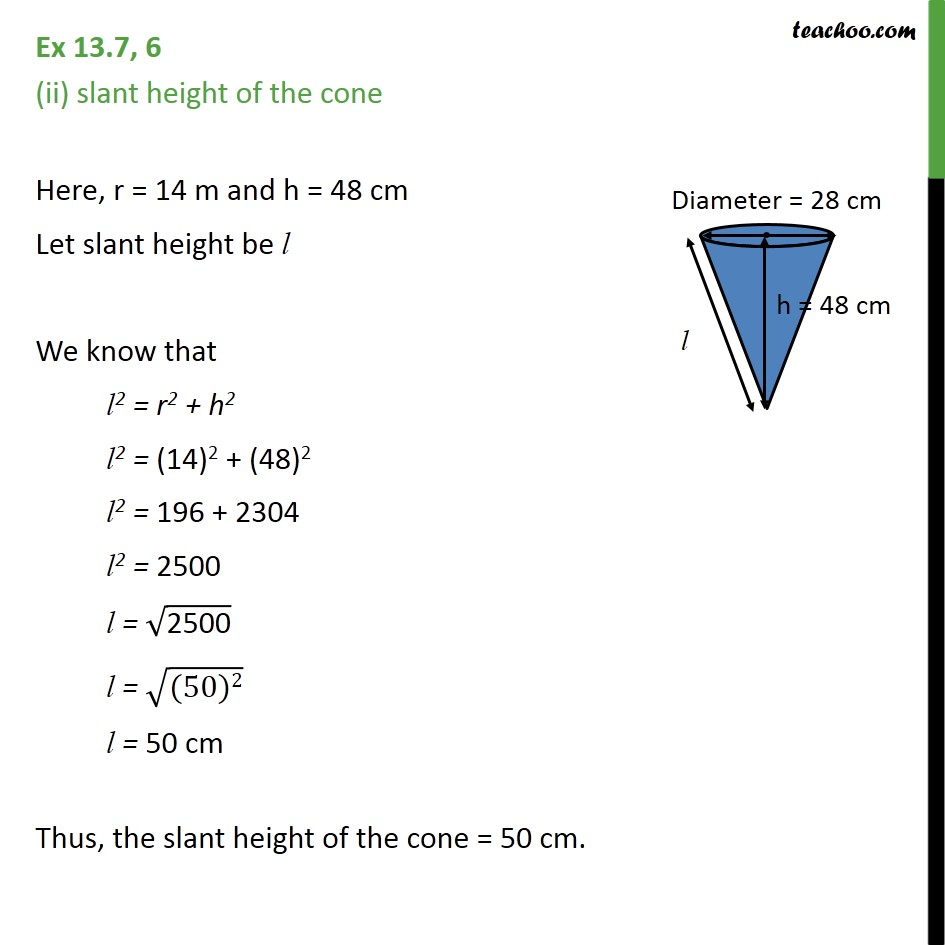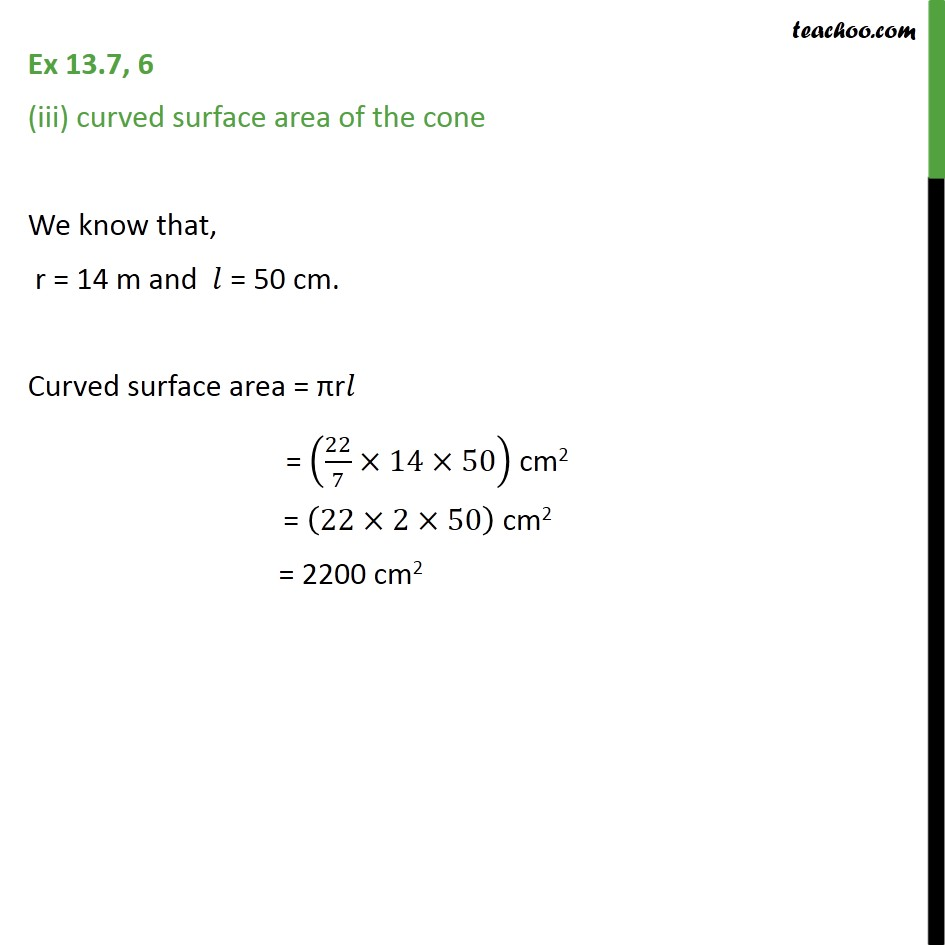Chapter 13 Class 9 Surface Areas and Volumes

Class 9
Important Questions for Exam - Class 9Learn in your speed, with individual attention - Teachoo Maths 1-on-1 Class

### Transcript

Ex 11.3, 6 The volume of a right circular cone is 9856 cm3. If the diameter of the base is 28 cm, find height of the cone Radius of cone = r = = cm = 14 cm Let Height of cone = h cm Volume of the cone = 9856 cm3 πr2h = 9856 = 9856 h = ∴ h = 48 Thus, the height of the cone = 48 cm. Ex 11.3, 6 (ii) slant height of the cone Here, r = 14 m and h = 48 cm Let slant height be l We know that l2 = r2 + h2 l2 = l2 = l2 = l = l = l = 50 cm Thus, the slant height of the cone = 50 cm. Ex 11.3, 6 (iii) curved surface area of the cone We know that, r = 14 m and = 50 cm. Curved surface area = πr = cm2 = cm2 = 2200 cm2